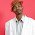## Sunday, June 20, 2010

### Python, Econometrics (fixed 06.21.2010): Wald test for Linear Equality Restrictions

Assume that the linear model $$Y = X B + u$$ is to be fitted by least squares for the unknown coefficients $$B$$. Then if the modeler may have some prior restriction(s) on the coefficients, then a Wald test may be applied to determine whether this restriction(s) is(are) statistically significant.

The restriction is written as a linear (matrix equation):
$$R_{(m \times k)} B_{(k \times 1)} = q_{(m x 1)}.$$

The test statistic is given by
$$W = (R\hat{\beta}-q)^t(\sigma^2R(X^t X)^{-1}R^t) ^{-1} (R \hat{\beta}-q)$$
where $$W$$ has a $$\chi^2(m)$$ distribution with m degrees of freedom and
standard table of that distribution can be used.
The Null hypothesis $$H_0: R\beta = q$$ is tested against the alternative hypothesis $$H_1: R\beta \ne q$$.

Our python function waldtest(R, q, fit) simply requires the user to input the R and q vectors as single dimensional Python arrays.The fit is the ols fitting object returned by the lm.ols function.

INSERT PYTHON FUNCTION HERE with example!!!

"""
"""
File   waldtest.py
Author Dr. Ernesto Adorio
U.P. Clark
ernesto.adorio@gmail.com
description
Computes the Wald statistic.
version 0.0.1 2010.06.20 first version
0.0.2 2010.06.21 fixed bugs for example.
"""

from matlib import *
from lm import *
from dataframe import *

def waldtest(R, q, fit):
"""
Performs a wald test on the linear equality restriction
equation RB = q.
R is a matrix of dimension m x k.
B is a vector of length k.
q is a vector of length m.
"""
m = len(R)
B = fit.betas
invxtx = fit.VC
sigmasqr = fit.MRSS # changed from fit.RSS, 06.21

# compute wald statistic!
m, k = matdim(R)
if m != len(q):
raise ValueError, "waldtest: R must have same rows as length of q!"
if k != len(B):
raise ValueError,"waldtest: R must have same columns as length of B!"
print "debug: sigmasqr=", sigmasqr
S  = matinverse(matkmul(matABAt(R, invxtx), sigmasqr)) # modified 06.21

RB = matvec(R,B)
RBmq = [rb - e for (rb,e) in zip(RB, q)]
print "debug:", RBmq

W  = matvectmatvec(RBmq, S)
return W, m

def Test():
"""
Danao's example page
"""
data = """
YEAR    OUTPUT  LABOR   CHEM    MACH
1947    58      297     15      54
1948    63      285     16      62
1949    62      285     18      68
1950    61      265     19      72
1951    63      251     21      77
1952    66      237     23      81
1953    66      220     24      82
1954    66      214     24      82
1955    69      220     26      83
1956    69      212     27      84
1957    67      196     27      83
1958    73      182     28      83
1959    74      183     32      84
1960    76      177     32      83
1961    76      167     35      80
1962    77      163     38      80
1963    80      155     43      79
1964    79      148     46      80
1965    82      144     49      80
1966    79      132     56      82
1967    83      128     66      85
1968    85      124     69      86
1969    85      118     73      86
1970    84      112     75      85
1971    92      108     81      87
1972    91      110     86      86
1973    93      109     90      90
1974    88      109     92      92
1975    95      106     83      96
1976    97      100     96      98
1977    100     100     100     100
1978    104     100     107     194
1979    111     99      123     104
1980    104     96      123     101
1981    118     96      129     98
1982    116     93      118     94
1983    96      97      105     90
1984    112     98      121     88
1985    113     84      123     83
"""
D = dataframe(data)
X = matSelectCols(D.data, [2,3,4])
matprint(X)

Xlog = []
for x in X:
Xlog.append([log(v) for v in x])
matInsertConstCol(Xlog, 0, 1.0)
matprint(Xlog)
Y = [log(y) for y in D.data]
fit = ols(Xlog, Y)
# print coefficients.
print "Coefficients:", fit.betas

R = [[0.,1.,1.,1.]]
q = 

W, m = waldtest(R, q, fit)
print "W statistic:", W

if __name__ == "__main__":
Test()


When the original 0.0.1 program was run, the output was

Coefficients: [2.4262377786012621, 0.10743484613669807, 0.33519197626861796, 0.029383835786802592]
W statistic: 0.57599916366


The coefficients match those of Danao, pp. 210, all right. But the W statistic .576 does not match the published value of 4.722226 So we will be in debug mode until we get this right.

After a good sleep, we disccovered that
sigmasqr should be fit.MRSS and the expresion S should be inverted! After these corrections,
the program test outputs the expected 4.722226!

SAFE TO USE THE PYTHON CODE CODE for computing the Wald statistic. but please, ABSOLUTELY NO WARRANTIES.

Will revise the code shortly to return the p-value of the test. Or readers who know Python can help.
We shall use the scipy function to do it.

The file dataframe.py may be found in http://adorio-research.org/wordpress/?p=7834.
We will post the updated matlib.py library soon!

#### 1 comment:

1.Why does w-stats is one figure hence it is not corresponding the coefficients??

Tsitso